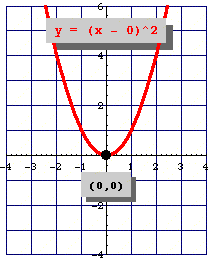# The Vertex of a Parabola

The vertex of a parabola is the point where the parabola crosses its axis of symmetry.   If the coefficient of the ${x}^{2}$ term is positive, the vertex will be the lowest point on the graph, the point at the bottom of the “ $U$ ”-shape.  If the coefficient of the ${x}^{2}$ term is negative, the vertex will be the highest point on the graph, the point at the top of the “ $U$ ”-shape.

The standard equation of a parabola is

$y=a{x}^{2}+bx+c$ .

But the equation for a parabola can also be written in "vertex form":

$y=a{\left(x-h\right)}^{2}+k$

In this equation, the vertex of the parabola is the point $\left(h,k\right)$ .You can see how this relates to the standard equation by multiplying it out:

$\begin{array}{l}y=a\left(x-h\right)\left(x-h\right)+k\\ y=a{x}^{2}-2ahx+a{h}^{2}+k\end{array}$ .

This means that in the standard form, $y=a{x}^{2}+bx+c$ , the expression $-\frac{b}{2a}$ gives the $x$ -coordinate of the vertex.

Example:

Find the vertex of the parabola.

$y=3{x}^{2}+12x-12$

Here, $a=3$ and $b=12$ . So, the $x$ -coordinate of the vertex is:

$-\frac{12}{2\left(3\right)}=-2$

Substituting in the original equation to get the $y$ -coordinate, we get:

$\begin{array}{l}y=3{\left(-2\right)}^{2}+12\left(-2\right)-12\\ \text{\hspace{0.17em}}\text{\hspace{0.17em}}\text{\hspace{0.17em}}\text{\hspace{0.17em}}=-24\end{array}$

So, the vertex of the parabola is at $\left(-2,-24\right)$ .